Courses
Courses for Kids
Free study material
Free LIVE classes
More# Classify the angles whose magnitudes are given below:$91^\circ$

Last updated date: 20th Mar 2023
Total views: 204k
Views today: 5.83kVerified
204k+ views
Hint: When two rays intersect each other and form an angle between $0^\circ$ to $90^\circ$, we can say that the angle between them is acute.
When two rays intersect each other and the angle between them is $90^\circ$, we can say that the angle between them is the right angle.
When two rays intersect each other and the angle between them is more than $90^\circ$ and less than $180^\circ$, then the angle is said to be an obtuse angle.

Complete step-by-step solution:
In this question, we are given a measure of an angle and we need to find out which type of angle it is.
Now, angles are the base of geometry and there are 4 types of angles in geometry.
Acute Angle:
When two rays intersect each other and form an angle between $0^\circ$ to $90^\circ$, we can say that the angle between them is acute.
For example: $30^\circ ,45^\circ ,60^\circ$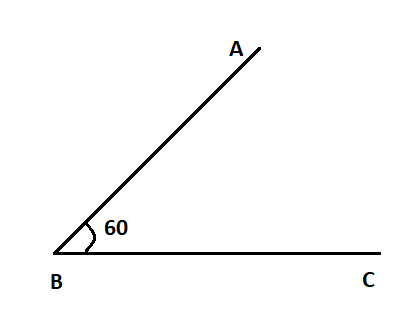Right angle:
When two rays intersect each other and the angle between them is $90^\circ$, we can say that the angle between them is the right angle.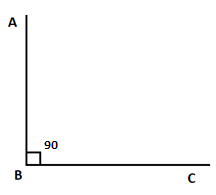Obtuse angles:
When two rays intersect each other and the angle between them is more than $90^\circ$ and less than $180^\circ$, then the angle is said to be obtuse angle.
For example: $95^\circ ,120^\circ ,130^\circ ,150^\circ$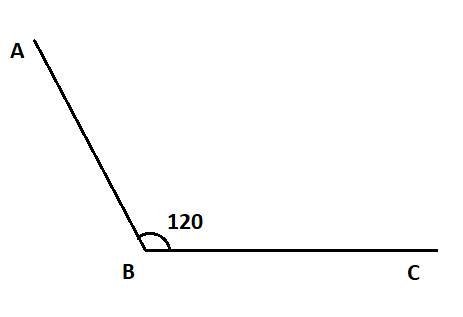Straight angle:
When the angle between two rays is equal to $180^\circ$, then we can say that the angle is straight angle.
For example: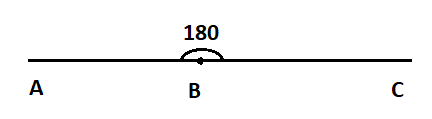Now, in our question, we are given an angle with measure $91^\circ$ which is greater than $90^\circ$ and less than $180^\circ$. Therefore, the given angle is obtuse angle.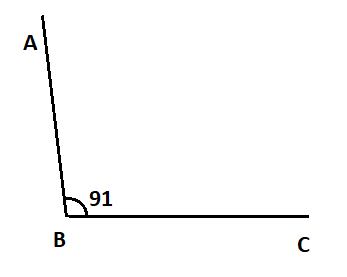Note: FOr answering this type of question we should have a theoretical concept of angles.
> When the sum measure of two angles equals to $90^\circ$, then the two angles are said to be complementary angles.
> When the sum measure of two angles equals to $180^\circ$, then the two angles are said to be supplementary angles.